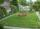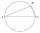# The area of triangle problems

#### Number of problems found: 337

• Area of a triangleWhat is the area of a triangle that has the base 4 1/4 and the height of 3 3/3?
• Is right triangle or notIf right triangle ABC, have sides a=13, b=11.5, c=22.5. Find area.
• Mysterious areaThe trapezoid ABCD is given. Calculate its area if the area of the DBC triangle is 27 cm2.
• Right triangleRight triangle ABC with side a = 19 and the area S = 95. Calculate the length of the remaining sides.
• TriangleThe triangle has known all three sides: a=5.5 m, b=5.3 m, c= 7.8 m. Calculate area of ​this triangle.
• SquareCalculate the area of the square shape of the isosceles triangle with the arms 50m and the base 60m. How many tiles are used to pave the square if the area of one tile is 25 dm2?
• Right triangleRight triangle legs has lengths 630 mm and 411 dm. Calculate the area of this triangle.
• Triangle SAAThe triangle has one side long 71 m and its two internal angles is 60°. Calculate the perimeter and area of the triangle.
• Triangle KLBIt is given equilateral triangle ABC. From point L which is the midpoint of the side BC of the triangle it is drwn perpendicular to the side AB. Intersection of perpendicular and the side AB is point K. How many % of the area of the triangle ABC is area o
• Vertex pointsGiven the following points of a triangle: P(-12,6), Q(4,0), R(-8,-6). Graph the triangle. Find the triangle area.
• Triangle SSSCalculate perimeter and area of ​​a triangle ABC, if a=53, b=46 and c=40.
• SAS triangleThe triangle has two sides long 7 and 19 and included angle 36°. Calculate area of this triangle.
• TriangleCalculate the sides of the triangle if its area S = 630 and the second cathethus is shorter by 17.
• ISO Triangle V2The perimeter of RR triangle (isosceles) is 474 m, and the base is 48 m longer than the arms. Calculate the area of this triangle.
• Garden fenceThe garden has the shape of a rectangular triangle with an area of 96 square meters and a 16 m long one leg. How many meters of the fence need to be fenced?
• Equilateral triangleCalculate the area of an equilateral triangle with circumference 72cm.
• Isosceles right triangleArea of an isosceles right triangle is 18 dm2. Calculate the length of its base.
• TriangleCalculate heights of the triangle ABC if sides of the triangle are a=75, b=84 and c=33.
• TriangleCalculate the area of right triangle ΔABC, if one leg is long 14 and its opposite angle is 59°.
• Equilateral triangle v2Equilateral triangle has a perimeter 36 dm. What is its area?

Do you have an interesting mathematical word problem that you can't solve it? Submit a math problem, and we can try to solve it.

We will send a solution to your e-mail address. Solved examples are also published here. Please enter the e-mail correctly and check whether you don't have a full mailbox.

Please do not submit problems from current active competitions such as Mathematical Olympiad, correspondence seminars etc...

Try trigonometric triangle area calculator. See also our trigonometric triangle calculator. Examples of area of plane shapes. Triangle Problems.